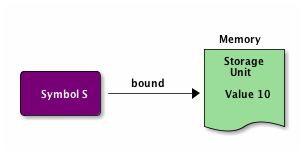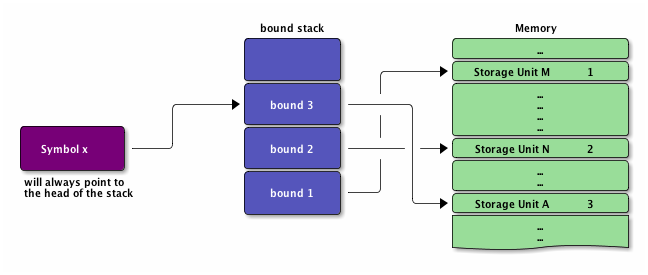Common Lisp是一种词法作用域（lexically scope）的Lisp，它同时支持两种类型的变量： 词法（lexical）变量动态（dynamic）变量 1

## （Common Lisp中）变量的基础知识

Lisp中所有符号只有两种状态：绑定和未绑定。4我们定义一个变量的同时，也建立了一个绑定，例如：

```CL-USER> (setf S 10)
10
CL-USER> S
10
```1. 把符号 `S` 跟内存中的一存储单元建立起绑定。之后，符号 `S` 出现的地方就代表（引用）了这一存储单元。
2. 把符号 `S` 所引用的存储单元的值设为整数10。我们接着在命令行中敲入 `S` 求值时，REPL会依据之前建立起的绑定找到那个存储单元并把值打印出来。

Common Lisp中所有的值，至少从概念上讲，都是对对象的引用（references）。5将一个变量赋予新值只会改变此变量将引用哪个对象，对其之前引用的对象没有影响。但是，如果一个变量保存了对一个可变对象的引用，你可以用这个引用来修改此对象，而且这种改动将作用于其他引用这个相同对象的代码。6

### 函数形参

```(defun foo (x y z) (+ x y z))
```

### LET 形式

```(let (variable*)
body-form*)
```

```(let ((x 10) (y 20) z)
...)
```

### 绑定形式的作用域

```(defun foo (x)
(format t "Parameter: ~a~%" x)      ; |<------ x为实参
(let ((x 2))                        ; |
(format t "Outer LET: ~a~%" x)    ; | |<---- x对应的值为2
(let ((x 3))                      ; | |
(format t "Inner LET: ~a~%" x)) ; | | |<-- x对应的值3
(format t "Outer LET: ~a~%" x))   ; | |
(format t "Parameter: ~a~%" x))     ; |
```

```CL-USER> (foo 1)
Parameter: 1
Outer LET: 2
Inner LET: 3
Outer LET: 2
Parameter: 1
NIL
```### 其他绑定形式

```(dotimes (x 10) (format t "~d " x))
```

```(let* ((x 10)
(y (+ x 10)))
(list x y))
```

```(let ((x 10)
(y (+ x 10)))
(list x y))
```

```(let ((x 10))
(let ((y (+ x 10)))
(list x y)))
```

## 词法变量和闭包

Common Lisp中将词法作用域和嵌套函数结合在一起时，按照词法作用域的规则，只有文本上位于绑定形式之内的代码可以引用词法变量，但是如果一个匿名函数包含了一个对于封闭区域内的词法变量的引用，例如下面的表达式：

```(let ((count 0))
#'(lambda ()
(setf count (1+ count))))
```

```(defparameter *fn* (let ((count 0))
#'(lambda ()
(setf count (1+ count)))))
```

```CL-USER> (funcall *fn*)
1
CL-USER> (funcall *fn*)
2
CL-USER> (funcall *fn*)
3
```

```(let ((count 0))
(list
#'(lambda () (incf count))
#'(lambda () (decf count))
#'(lambda () count)))
```

## 动态变量（Dynamic, a.k.a. Special, Variables）

Common Lisp提供两种方式来创建全局变量： DEFVARDEFPARAMETER 。两种方式都接受一个变量名，一个初始值和一个可选的文档字符串。在被 DEFVARDEFPARAMETER 定义之后，该变量名将可以在任何地方使用来引用全局变量的当前绑定。全局变量习惯上被命名为以 * 开始和结束，遵守该命名约定非常重要。 DEFVARDEFPARAMETER 的示例如下：

```(defvar *count* 0
"Count of widgets made so far.")

(defparameter *gap-tolerance* 0.001
"Tolerance to be allowed in widget gaps.")
```

```(defun increment-widget-count ()
(incf *count*))
```

```(defvar *x* 10)

(defun foo ()
(format t "X: ~d~%" *x*))
```

```CL-USER> (foo)
X: 10
NIL
```

```CL-USER> (let ((*x* 20)) (foo))
X: 20
NIL
```

```CL-USER> (foo)
X: 10
NIL
```

```(defun bar ()
(foo)
(let ((*x* 20)) (foo))
(foo))
```

```CL-USER> (bar)
X: 10
X: 20
X: 10
NIL
```

```(defun foo ()
(format t "Before assignment~18tX: ~d~%" *x*)
(setf *x* (+ 1 *x*))
(format t "After assignment~18tX: ~d~%" *x*))
```

```CL-USER> (foo)
Before assignment X: 10
After assignment  X: 11
NIL
```

```CL-USER> (bar)
Before assignment X: 11
After assignment  X: 12
Before assignment X: 20
After assignment  X: 21
Before assignment X: 12
After assignment  X: 13
NIL
```

## 其他参考来源

1. 《实用Common Lisp编程》－人民邮电出版社，第1版，田春译
2. 《On Lisp中文版》 － 田春等译
3. 静态绑定和动态绑定
4. Common Lisp 变量作用域

## Footnotes:

1

2

3

4

5

6

7

Common Lisp函数是“传值的”，但这些被传递的值是对对象的引用。这跟Java和Python的工作方式相似。

8

9

10

11

LET 形式和函数形参中的变量是以完全相同的机制创建的。事实上，在某些Lisp方言中，不是Common Lisp， LET 只是一个展开到一个匿名函数调用的宏。也就是说，在那些方言中， `(let ((x 10)) (format t "~a" x))` 是一个展开到下列结果的宏： `((lambda (x) (format t "~a" x)) 10)`

12

13

14

15

16

17